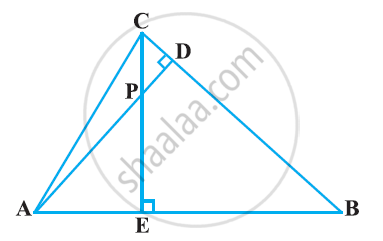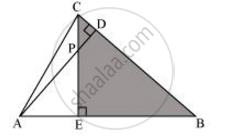# In the following figure, altitudes AD and CE of ΔABC intersect each other at the point P. Show that - Mathematics

In the following figure, altitudes AD and CE of ΔABC intersect each other at the point P. Show that:ΔPDC ∼ ΔBEC

#### SolutionIn ΔPDC and ΔBEC,

∠PDC = ∠BEC (Each 90°)

∠PCD = ∠BCE (Common angle)

Hence, by using AA similarity criterion,

ΔPDC ∼ ΔBEC

Concept: Criteria for Similarity of Triangles
Is there an error in this question or solution?

#### APPEARS IN

NCERT Class 10 Maths
Chapter 6 Triangles
Exercise 6.3 | Q 7.4 | Page 140